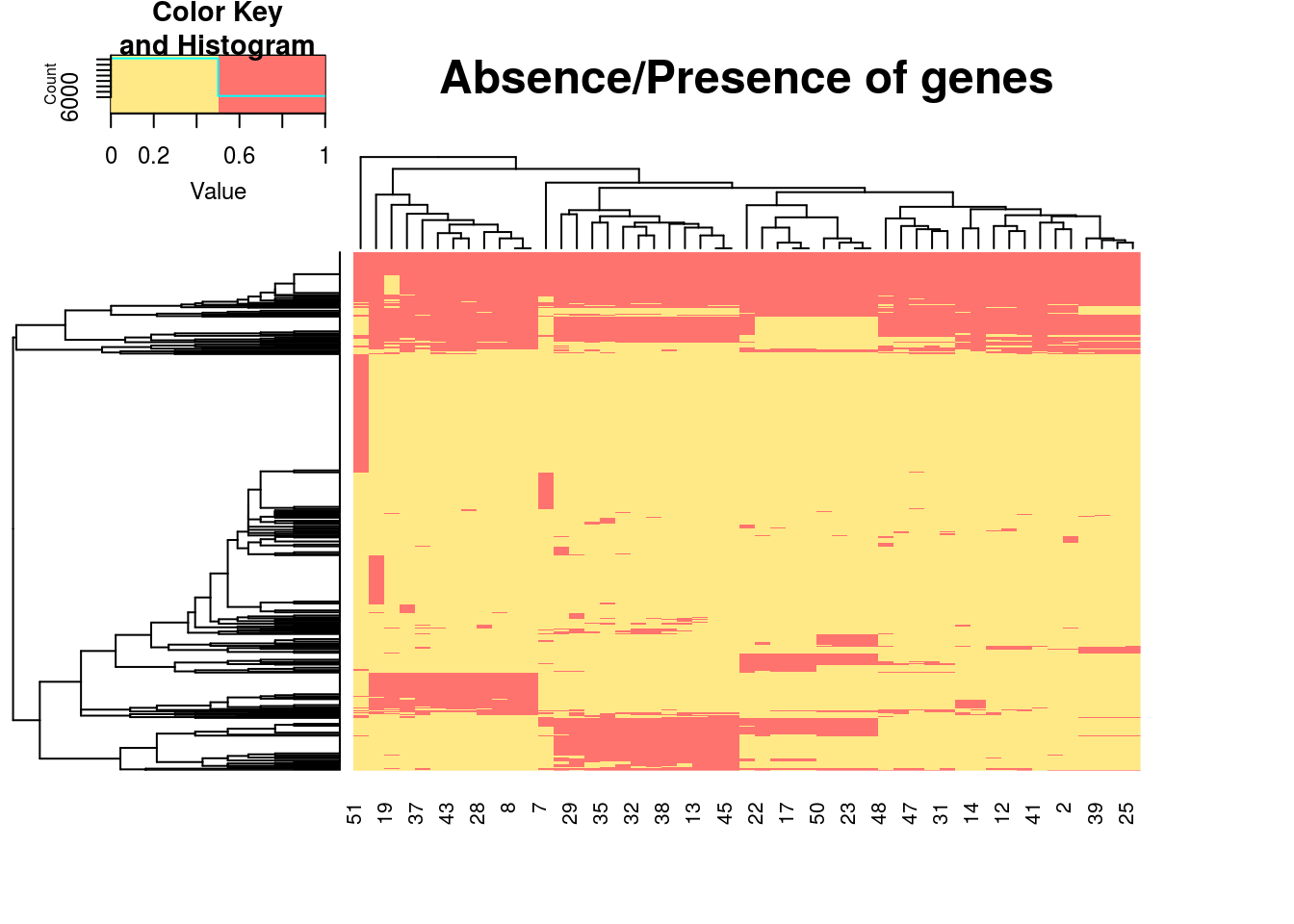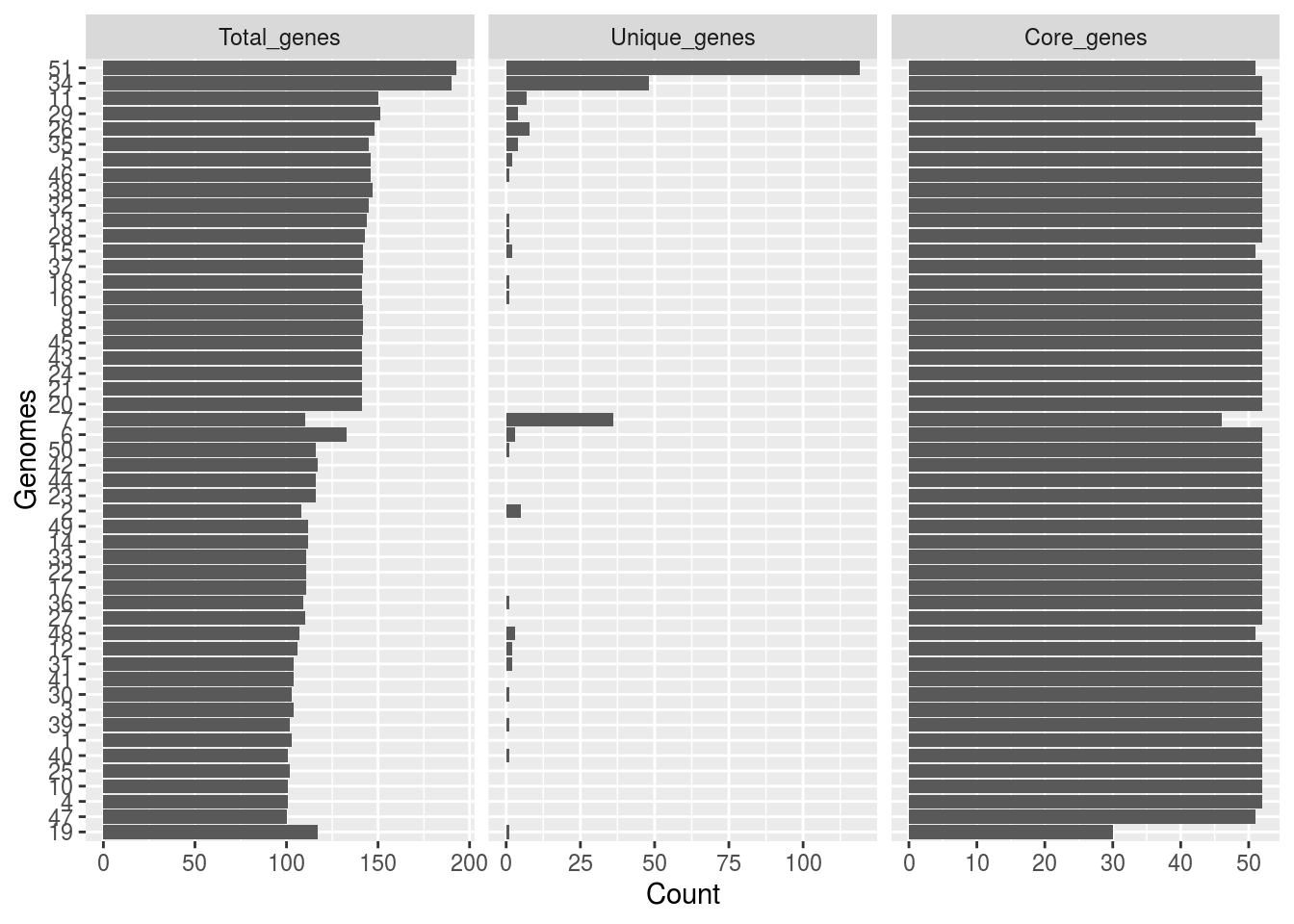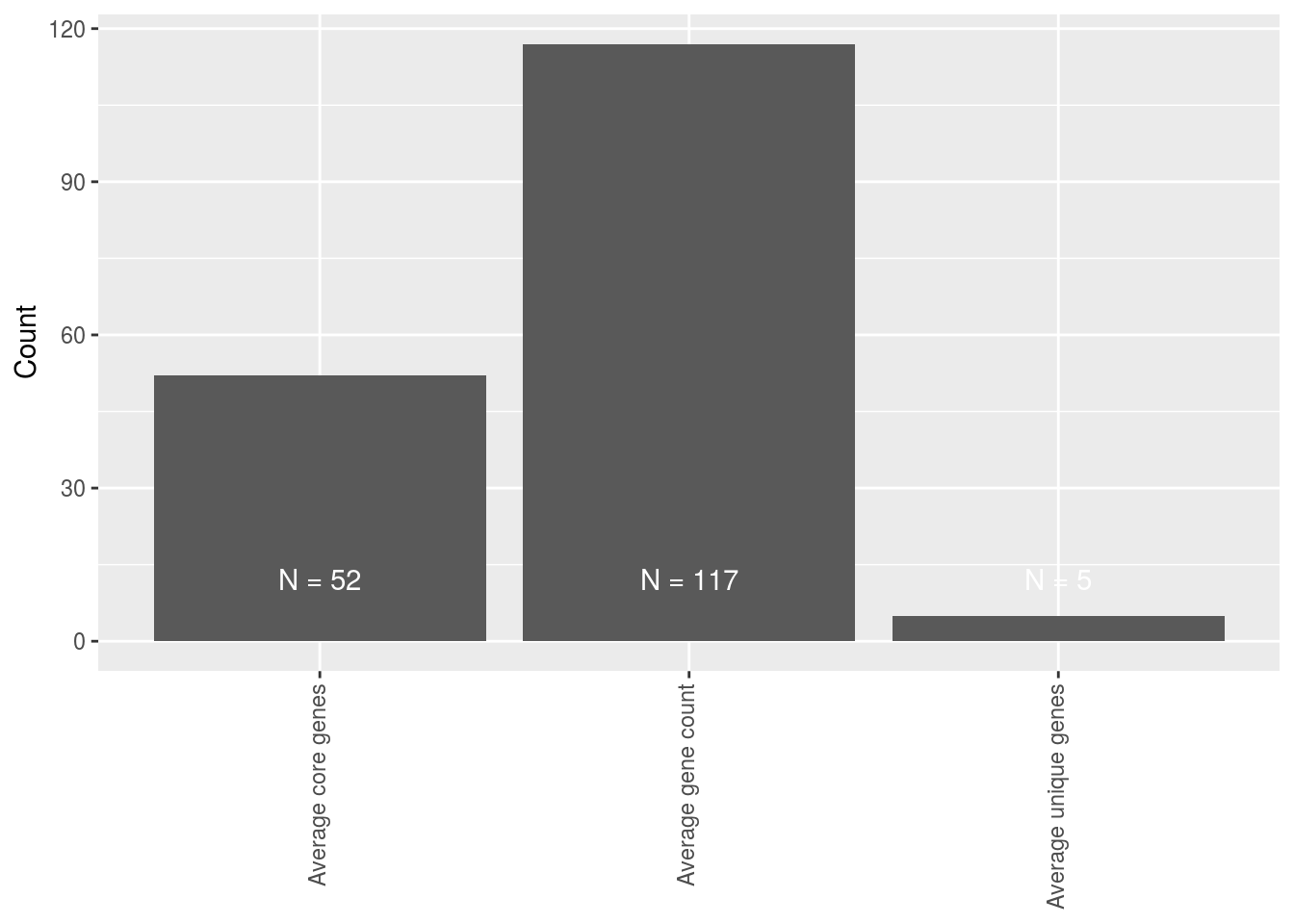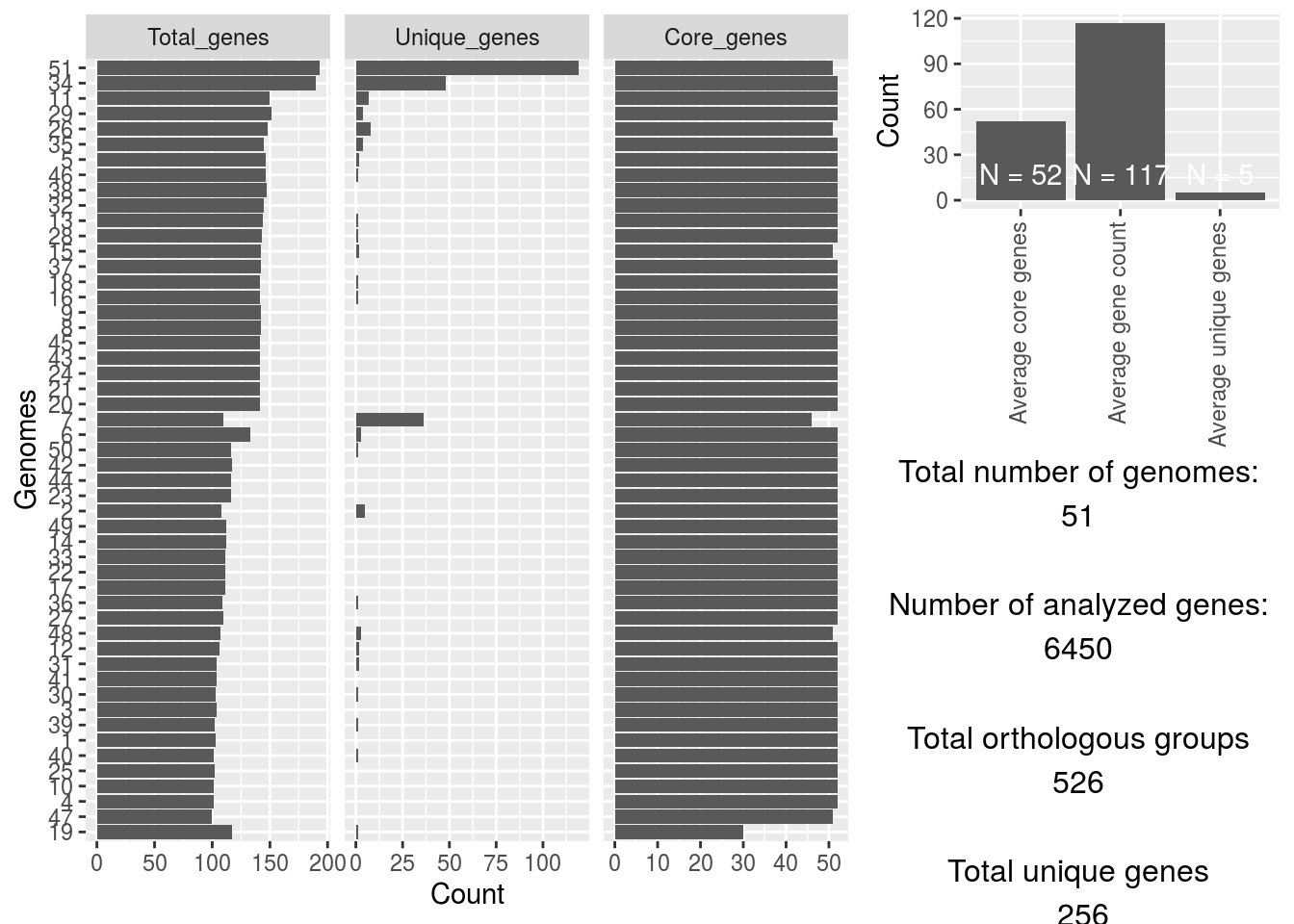THOMAS LESLEY SITTER

Bioinformatician / Microbiologist / All round geek# Roary analysis

Data processing

For my work i work with genomic data and one tool i enjoy using is Roary. Roary is a orthologous gene clustering tool that uses BLAST to find similar genes, and if their similarity is above a threshold it would consider them being orthologous. In my case i normally stick to a 90% cutoff.

The output of roary is a huge excell sheet with genes and it also gives some plots about the amount of unique genes per genome, amount of new genes the genome adds to the total pool, and some other stats. The problem is that it’s not outputted in a way users can easily understand, they randomly sample the datasets so their data works as some generic output, but is totally uninformative. So here we’ll convert Roary to a nice looking plot, and also output some summary stats.

First off we’ll load our randomly stolen packages found on stackoverflow that is slightly changed to my liking. (If this is based on your code, please send me the stackoverflow link and i’ll add it)

detachAllPackages <- function() {
basic.packages <- c("package:stats","package:graphics","package:grDevices","package:utils","package:datasets","package:methods","package:base")
package.list <- search()[ifelse(unlist(gregexpr("package:",search()))==1,TRUE,FALSE)]
package.list <- setdiff(package.list,basic.packages)
if (length(package.list)>0)  for (package in package.list) detach(package, character.only=TRUE)
}

detachAllPackages()

for(package in Required_Packages){
if (!package %in% installed.packages()) install.packages(package, character.only = TRUE)
library(package, character.only = TRUE)
}
}

We’ll use a number of packages for this project. We need gplots for our plots, colorspace for some color changes and dplyr for some data manipulations.

Now we’ll load the data and convert it into a data frame

table_input <- read.csv("Roary_gene_presence_absence.csv", sep=",", na.strings=c("","NA"))
table_input <- as.data.frame(table_input)

The ouput of Roary looks like this, with p1 being our first actually datapoint.

Because Roary outputs a lot of data as you can see below, we’ll omit most of it, and only focus on the absence/presence matrix part of it.

table_values <- within(table_input, rm("Gene","Non.unique.Gene.name","No..isolates","No..sequences","Avg.sequences.per.isolate","Genome.Fragment","Order.within.Fragment","Accessory.Fragment","Accessory.Order.with.Fragment","QC","Min.group.size.nuc","Max.group.size.nuc","Avg.group.size.nuc"))

Now we don’t need the data.frame portion anymore, we’ll convert the data to a matrix as this makes plotting easier and we’ll change the absence/presence matrix to a binary matrix.

abscence_presence <- as.matrix(table_values[,-1])
rownames(abscence_presence) <- table_values[,1]
abscence_presence[is.na(abscence_presence)] <- 0
abscence_presence[which(abscence_presence!=0)] <- 1

For some magical R reason some values aren’t numerical… instead of figuring out the reason, i’ll just go ahead and use mapply to convert each value in our binary matrix to a numeric value.

a_p_matrix <- mapply(abscence_presence, FUN=as.numeric)
a_p_matrix <- matrix(data=a_p_matrix, ncol=length(colnames(abscence_presence)), nrow=length(row.names(abscence_presence)))
row.names(a_p_matrix) <- row.names(abscence_presence)
colnames(a_p_matrix) <- colnames(abscence_presence)

Now we have a nice binary matrix to work from. Using heatmap.2 we can nicely plot this table into a heatmap, and let the the function cluster similar paterns together so we won’t have to. And we’ll use labRow=FALSE to supress all row labels as there are +/- 600 genes, so not really informative for this example.

heatmap.2(a_p_matrix, col = c("#FFE986","#FF736E"), main = "Absence/Presence of genes", trace="none", labRow=FALSE)Now that we have our nice heatmap, we also want some of that sweet sweet summary data. We’ll add the total number of dataset analyzed as we’ll be using it a couple times further on.

genomes_count <- length(colnames(a_p_matrix))

How we’ll do this is by adding a summary column at the end… In this example all the othrlog groups are on the rows, so this way we can easily calculate how many genomes have gene X, by just summarizing the binary matrix into this column.

abscence_presence <- cbind(a_p_matrix, rowSums(a_p_matrix))

Now to not further tamper with our matrix, we’ll create a new matrix, that has three columns per dataset, one for total amount of genes that dataset has, one for the total amount of unique genes that dataset has, and one that will see how many genes are present in the majority of the strains based on some hardcoded cuttoff which we’ll get to later.

summary_table <- matrix(data=NA, nrow=3, ncol=length(colnames(abscence_presence)))
colnames(summary_table) <- colnames(abscence_presence)
rownames(summary_table) <- c("Total_genes","Unique_genes","Core_genes")

We’ll first calculate the sum of all columns in our absence presence matrix, this will give us the “total genes” per dataset.

summary_table[1,] <- colSums(abscence_presence)

Based on the values in our summary column we can now subset our data and only grab the “unique genes” based on groups that only have 1 in the summary.

summary_table[2,] <- colSums(abscence_presence[which(abscence_presence[,ncol(abscence_presence)] == 1),])

We can subset our data to extract the “CORE genes” by taking all the rows containing more than 1 and applying some cuttoff. In this case we’ll go with 0.95 (95%) of genomes_count (total), i.e. 95% of the total datasets have to have this gene to consider it a “CORE gene”.

summary_table[3,] <- colSums(abscence_presence[which(abscence_presence[,ncol(abscence_presence)] >= (genomes_count*0.95)),])

This code was a bit sloppy in that it also analyzed the summary column and stored that in our summary table. This column doesn’t contain usable data, so we’ll just remove it

summary_table <- summary_table[,-ncol(summary_table)]

No that we have all these stats, we’ll do some other stats as well that are more about the whole Roary analysis and less about individual datasets. We’ll call it average_table.

average_table <- data.frame(x=1:6, y=1:6, z=1:6)

We will calculate some stats such as total genes analyzed, total orthologous groups, average gene count per dataset, average core genes, average unique genes and total unique genes. We could add more by simply performing some calculations over our summary_table and absence_presence matrix.

average_table[,1] <- c("Total genes analyzed","Orthologous groups","Average gene count","Average core genes","Average unique genes","Total unique genes")
average_table[1,2] <- sum(summary_table[1,])
average_table[2,2] <- length(rownames(abscence_presence))
average_table[3,2] <- median(summary_table[1,])
average_table[4,2] <- length(rownames(abscence_presence[which(abscence_presence[,ncol(abscence_presence)] >= (genomes_count*0.95)),]))
average_table[5,2] <- round(length(rownames(abscence_presence[which(abscence_presence[,ncol(abscence_presence)] == 1),]))/length(colnames(abscence_presence)))
average_table[6,2] <- length(rownames(abscence_presence[which(abscence_presence[,ncol(abscence_presence)] == 1),]))

Now ggplot doesn’t enjoy regular square matrixes as much as it likes long form matrixes, so we’ll use melt from reshape2 and while we’re at it we’ll plot the data using ggplot2, and we’ll use grid and gridExtra to put several of the plots into one graphical device.

Now we can use melt on the summary table, and we’ll order the plots from highest amount of genes to lowest

melt_summary_table <- melt(summary_table)
melt_summary_table <- melt_summary_table[order(melt_summary_table\$value),]

First plot is the plot for total_genes per genome, total unique genes per genome and total “core” genes per genome. It’s a flipped bar chart, if you want anything else just change geom_bar into geom_line or geom_anythingelse

p1 <- ggplot(melt_summary_table, aes(x = reorder(Var2, value), y = value)) +
geom_bar( stat = 'identity') +
facet_grid(. ~ Var1, scales = "free_x") +
xlab("Genomes") +
ylab("Count") +
coord_flip()Second plot is for the “averages” so it shows average number of genes, average number of unique genes, and average number of core genes. Could be handy for outliers, shows how much each dataset deviates from the norm

p2 <- ggplot(data=average_table[-c(1,2,6),], aes(x=x, y=y))+
geom_bar(stat = 'identity') +
theme (axis.text.x=element_text(angle=90,hjust=1,vjust=0.3),
axis.title.x = element_blank()) +
geom_text(aes(y = 10, label = paste("N =" ,y),vjust = 0), colour = "white", show.legend=FALSE) +
ylab("Count")And a little text box giving some Roary output information such as total numer of datasets, total number of genes etc.

t1  <- textGrob(paste(c("Total number of genomes:\n",
length(colnames(summary_table)),
"\n\nNumber of analyzed genes:\n",
as.numeric(average_table[1,2]),
"\n\nTotal orthologous groups\n",
as.numeric(average_table[2,2]),
"\n\nTotal unique genes\n",
as.numeric(average_table[6,2])), collapse = " ")) 
## text[GRID.text.148]

Now we have our plots stored in p1, p2 and t1, we’ll have to figure out how we want to show it. The biggest plot is the p1 plot as it has 3 types of information for all dataset, so essentially 3 plots into on. The p2 plot is a small plot consists of a single barchart that only has a couple values, and the t1 textbox only has 8 lines of text. We’ll use rbind to make a matrix that shows how much space each plot gest

lay <- rbind(c(1,1,2),
c(1,1,2),
c(1,1,3),
c(1,1,3))

And now all that is left is plotting it.

grid.arrange(p1,p2,t1, layout_matrix = lay)Thank you for flying Lesley Airlines!

### 2 thoughts on “Roary analysis”

1.Excelent tutorial! Work perfect!

1.Awesome 😀 and additionally, the output is in pdf, therefore everything in the PDF are vectors that you can edit with tools like InkScape, CorelDraw, Adobe Illustrator… that way you can easily highlight labels, removes axis, change colors etc if it’s too hard to do through R 😀